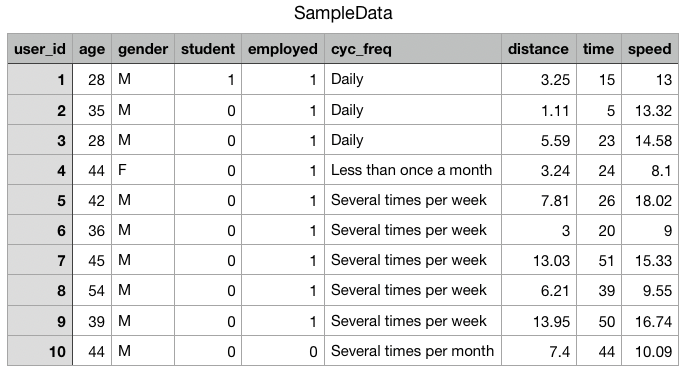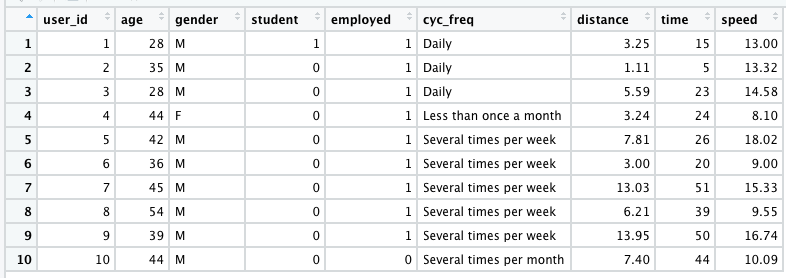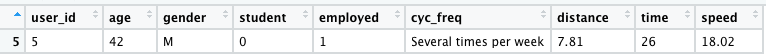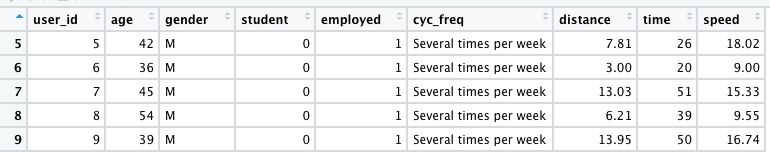# Reading the CSV file into Dataframes in R

• Last Updated : 09 May, 2021

In this article, we will learn how to import or read a CSV file into a dataframe in R Programming Language.

Data set in use:### Step 1: Set or change the working directory

In order to import or read the given CSV file into our data frame, we first need to check our current working directory, and make sure that the CSV file is in the same directory as our R studio is in, or else it might show “File not found Error”.

To check the current working directory we need to use getwd() function, and to change the current working directory to some other working directory, we need to use stewd() function.

getwd() returns an absolute file-path representing the current working directory of the R process.

Syntax:

getwd()

setwd(dir) used to set the working directory to dir.

Syntax:

setwd(path)

Example:

## R

 `# gives the current working directory``getwd``()`` ` `# changes the location ``setwd``(``"C:/Users/Vanshi/Desktop/gfg"``)`

Output:

C:/Users/Vanshi/Documents

### Step 2: Read the CSV file

Now that we have set our working path, we will import the CSV file into the data frame, and name our data frame as sdata.

Here, we are reading the .csv file named “SampleData” using read.csv command, into our R studio, which means we are feeding the values to the Rstudio to extract some important information out of it.

read.csv() function reads a file in table format and creates a data frame from it, with cases corresponding to lines and variables to fields in the file.

Syntax: read.csv(file, header = TRUE, sep = “,”, quote = “\””, dec = “.”, fill = TRUE, comment.char = “”, …)

Arguments:

• file: the name of the file which the data are to be read from.
• header: a logical value indicating whether the file contains the names of the variables as its first line. If missing, the value is determined from the file format: header is set to TRUE if and only if the first row contains one fewer field than the number of columns.
• sep: the field separator character. Values on each line of the file are separated by this character. If sep = “” (the default for read.table) the separator is ‘white space’, that is one or more spaces, tabs, newlines or carriage returns.
• quote: the set of quoting characters.
• dec: the character used in the file for decimal points.
• fill: logical. If TRUE then in case the rows have unequal length, blank fields are implicitly added.
• comment.char: character: a character vector of length one containing a single character or an empty string.
• … :  Further arguments to be passed.

Example:

## R

 `sdata <- ``read.csv``(``"SampleData.csv"``, header = ``TRUE``, sep = ``","``)``sdata`` ` `# views the data frame formed from the csv file``View``(sdata)`

Output:Now that, we have created our dataframe, we can perform some operations on it. The data read according to the usage from dataframe. Given below are two examples who read the data as per their requirement.

Example 1:

## R

 `sdata <- ``read.csv``(``  ``"SampleData.csv"``, header = ``TRUE``, sep = ``","``)`` ` `highspeed <- ``subset``(``  ``sdata, sdata\$speed == ``max``(sdata\$speed))`` ` `# views the subsetted value in ``# tabular form``View``(highspeed)`

Output:Example 2:

## R

 `sdata <- ``read.csv``(``  ``"SampleData.csv"``, header = ``TRUE``, sep = ``","``)`` ` `highfreq <- ``subset``(``  ``sdata, sdata\$cyc_freq == ``"Several times per week"``)`` ` `# views the information, of the above ``# condition in tabular format``View``(highfreq)`

Output:My Personal Notes arrow_drop_up